## Solve the system of inequalities to decide if the point (-3,2) is part of the solution; y<=-4x-3, +8y>=7

Question

Solve the system of inequalities to decide if the point (-3,2) is part of the solution; y<=-4x-3, +8y>=7

in progress 0
6 months 2021-07-30T00:13:11+00:00 1 Answers 2 views 0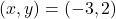is a solution

Step-by-step explanation:

Given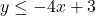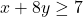Required

Determine ifis a solutionbecomes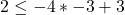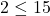— this is true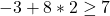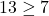— this is also true

Hence,is a solution